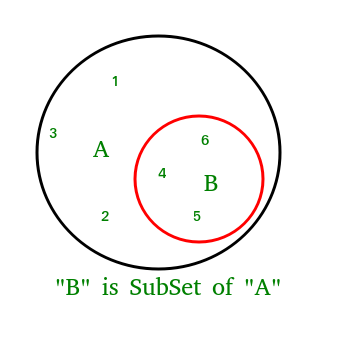# issubset() in python

• Difficulty Level : Basic
• Last Updated : 05 Oct, 2021

Python set issubset() method returns True if all elements of a set A are present in another set B which is passed as an argument and returns false if all elements not present.

### Python set issubset() Syntax:

```A.issubset(B)
checks whether A is a subset of B or not.```

### Python set isubset() Return:

`returns true if A is a subset of B otherwise false.`Attention geek! Strengthen your foundations with the Python Programming Foundation Course and learn the basics.

To begin with, your interview preparations Enhance your Data Structures concepts with the Python DS Course. And to begin with your Machine Learning Journey, join the Machine Learning - Basic Level Course

## Python

 `# Python program to demonstrate working of``# issubset().` `A ``=` `{``4``, ``1``, ``3``, ``5``}``B ``=` `{``6``, ``0``, ``4``, ``1``, ``5``, ``0``, ``3``, ``5``}` `# Returns True``print``(A.issubset(B))` `# Returns False``# B is not subset of A``print``(B.issubset(A))`

Output:

```True
False```

## Python

 `# Another Python program to demonstrate working``# of issubset().``A ``=` `{``1``, ``2``, ``3``}``B ``=` `{``1``, ``2``, ``3``, ``4``, ``5``}``C ``=` `{``1``, ``2``, ``4``, ``5``}` `# Returns True``print``(A.issubset(B))` `# Returns False``# B is not subset of A``print``(B.issubset(A))` `# Returns False``print``(A.issubset(C))` `# Returns True``print``(C.issubset(B))`

Output:

```True
False
False
True```

My Personal Notes arrow_drop_up